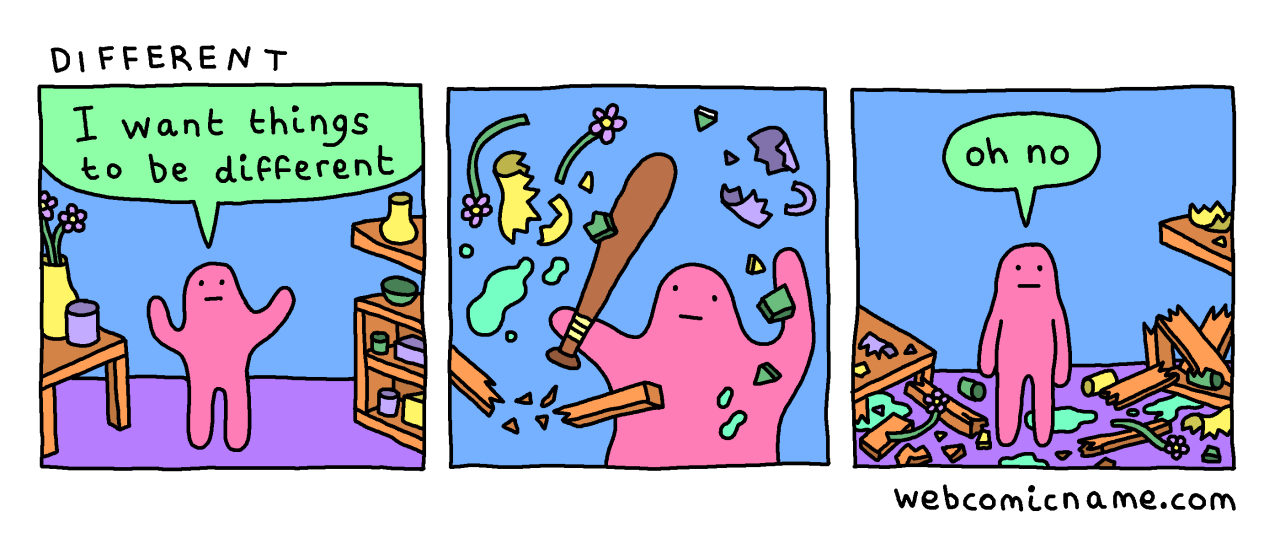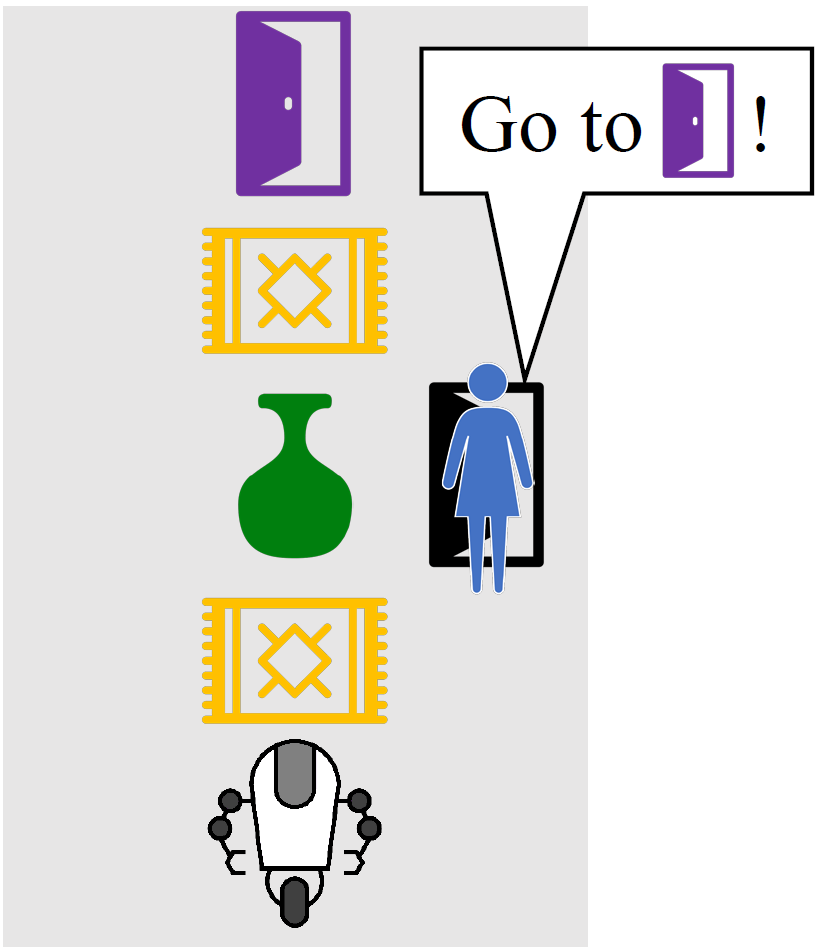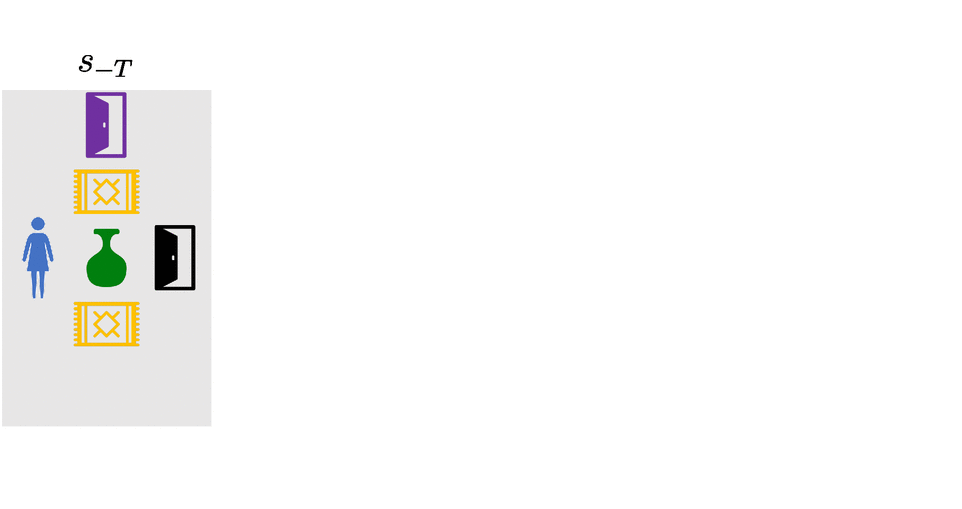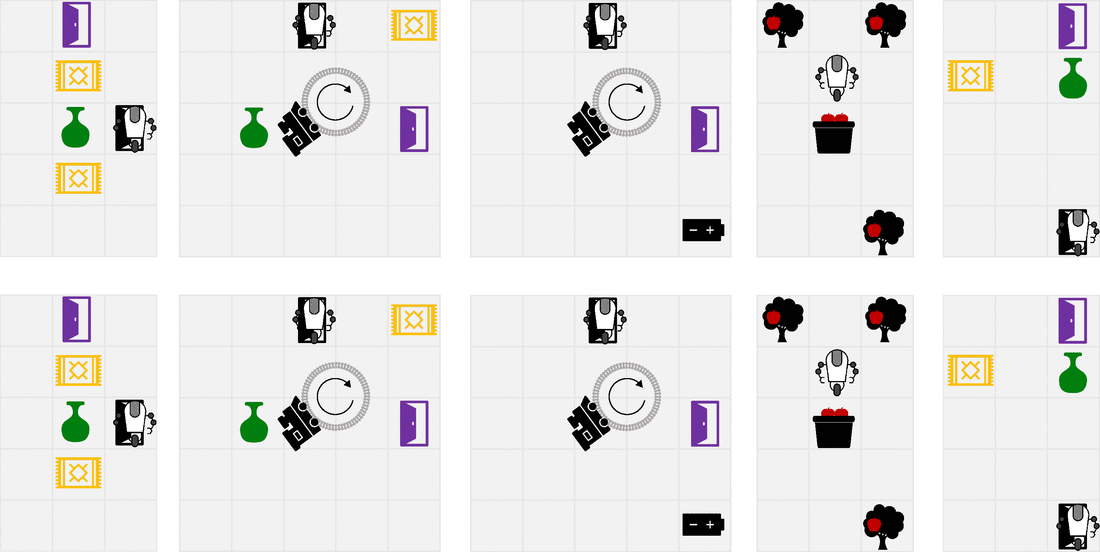news    views    podcast    learn    |    about    contribute     republish

## Learning preferences by looking at the world

February 12, 2019

By Rohin Shah and Dmitrii Krasheninnikov

It would be great if we could all have household robots do our chores for us. Chores are tasks that we want done to make our houses cater more to our preferences; they are a way in which we want our house to be different from the way it currently is. However, most “different” states are not very desirable:Surely our robot wouldn’t be so dumb as to go around breaking stuff when we ask it to clean our house? Unfortunately, AI systems trained with reinforcement learning only optimize features specified in the reward function and are indifferent to anything we might’ve inadvertently left out. Generally, it is easy to get the reward wrong by forgetting to include preferences for things that should stay the same, since we are so used to having these preferences satisfied, and there are so many of them. Consider the room below, and imagine that we want a robot waiter that serves people at the dining table efficiently. We might implement this using a reward function that provides 1 reward whenever the robot serves a dish, and use discounting so that the robot is incentivized to be efficient. What could go wrong with such a reward function? How would we need to modify the reward function to take this into account? Take a minute to think about it.Here’s an incomplete list we came up with:

• The robot might track dirt and oil onto the pristine furniture while serving food, even if it could clean itself up, because there’s no reason to clean but there is a reason to hurry.
• In its hurry to deliver dishes, the robot might knock over the cabinet of wine bottles, or slide plates to people and knock over the glasses.
• In case of an emergency, such as the electricity going out, we don’t want the robot to keep trying to serve dishes – it should at least be out of the way, if not trying to help us.
• The robot may serve empty or incomplete dishes, dishes that no one at the table wants, or even split apart dishes into smaller dishes so there are more of them.

Note that we’re not talking about problems with robustness and distributional shift: while those problems are worth tackling, the point is that even if we achieve robustness, the simple reward function still incentivizes the above unwanted behaviors.

It’s common to hear the informal solution that the robot should try to minimize its impact on the environment, while still accomplishing the task. This could potentially allow us to avoid the first three problems above, though the last one still remains as an example of specification gaming. This idea leads to impact measures that attempt to quantify the “impact” that an agent has, typically by looking at the difference between what actually happened and what would have happened had the robot done nothing. However, this also penalizes things we want the robot to do. For example, if we ask our robot to get us coffee, it might buy coffee rather than making coffee itself, because that would have “impact” on the water, the coffee maker, etc. Ultimately, we’d like to only prevent negative impacts, which means that we need our AI to have a better idea of what the right reward function is.

Our key insight is that while it might be hard for humans to make their preferences explicit, some preferences are implicit in the way the world looks: the world state is a result of humans having acted to optimize their preferences. This explains why we often want the robot to by default “do nothing” – if we have already optimized the world state for our preferences, then most ways of changing it will be bad, and so doing nothing will often (though not always) be one of the better options available to the robot.

Since the world state is a result of optimization for human preferences, we should be able to use that state to infer what humans care about. For example, we surely don’t want dirty floors in our pristine room; otherwise we would have done that ourselves. We also can’t be indifferent to dirty floors, because then at some point we would have walked around the room with dirty shoes and gotten a dirty floor. The only explanation is that we want the floor to be clean.

# A simple setting

Let’s see if we can apply this insight in the simplest possible setting: gridworlds with a small number of states, a small number of actions, a known dynamics model (i.e. a model of “how the world works”), but an incorrect reward function. This is a simple enough setting that our robot understands all of the consequences of its actions. Nevertheless, the problem remains: while the robot understands what will happen, it still cannot distinguish good consequences from bad ones, since its reward function is incorrect. In these simple environments, it’s easy to figure out what the correct reward function is, but this is infeasible in a real, complex environment.For example, consider the room to the right, where Alice asks her robot to navigate to the purple door. If we were to encode this as a reward function that only rewards the robot while it is at the purple door, the robot would take the shortest path to the purple door, knocking over and breaking the vase – since no one said it shouldn’t do that. The robot is perfectly aware that its plan causes it to break the vase, but by default it doesn’t realize that it shouldn’t break the vase.

In this environment, does it help us to realize that Alice was optimizing the state of the room for her preferences? Well, if Alice didn’t care about whether the vase was broken, she would have probably broken it some time in the past. If she wanted the vase broken, she definitely would have broken it some time in the past. So the only consistent explanation is that Alice cared about the vase being intact, as illustrated in the gif below.While this example has the robot infer that it shouldn’t take the action of breaking a vase, the robot can also infer goals that it should actively pursue. For example, if the robot observes a basket of apples near an apple tree, it can reasonably infer that Alice wants to harvest apples, since the apples didn’t walk into the basket themselves – Alice must have put effort into picking the apples and placing them in the basket.

# Reward Learning by Simulating the Past

We formalize this idea by considering an MDP in which our robot observes the initial state $s_0$ at deployment, and assumes that it is the result of a human optimizing some unknown reward for $T$ timesteps.

Before we get to our actual algorithm, consider a completely intractable algorithm that should do well: for each possible reward function, simulate the trajectories that Alice would take if she had that reward, and see if the resulting states are compatible with $s_0$. This set of compatible reward functions give the candidates for Alice’s reward function. This is the algorithm that we implicitly use in the gif above.

Intuitively, this works because:

• Anything that requires effort on Alice’s part (e.g. keeping a vase intact) will not happen for the vast majority of reward functions, and will force the reward functions to incentivize that behavior (e.g. by rewarding intact vases).
• Anything that does not require effort on Alice’s part (e.g. a vase becoming dusty) will happen for most reward functions, and so the inferred reward functions need not incentivize that behavior (e.g. there’s no particular value on dusty/clean vases).

Another way to think of it is that we can consider all possible past trajectories that are compatible with $s_0$, infer the reward function that makes those trajectories most likely, and keep those reward functions as plausible candidates, weighted by the number of past trajectories they explain. Such an algorithm should work for similar reasons. Phrased this way, it sounds like we want to use inverse reinforcement learning to infer rewards for every possible past trajectory, and aggregate the results. This is still intractable, but it turns out we can take this insight and turn it into a tractable algorithm.

We follow Maximum Causal Entropy Inverse Reinforcement Learning (MCEIRL), a commonly used algorithm for small MDPs. In this framework, we know the action space and dynamics of the MDP, as well as a set of good features of the state, and the reward is assumed to be linear in these features. In addition, the human is modelled as Boltzmann-rational: Alice’s probability of taking a particular action from a given state is assumed to be proportional to the exponent of the state-action value function Q, computed using soft value iteration. Given these assumptions, we can calculate $p(\tau \mid \theta_A)$, the distribution over the possible trajectories $\tau = s_{-T} a_{-T} \dots s_{-1} a_{-1} s_0$ under the assumption that Alice’s reward was $\theta_A$. MCEIRL then finds the $\theta_A$ that maximizes the probability of a set of trajectories $\{\tau_i\}$.

Rather than considering all possible trajectories and running MCEIRL on all of them to maximize each of their probabilities individually, we instead maximize the probability of the evidence that we see: the single state $s_0$. To get a distribution over $s_0$, we marginalize out the human’s behavior prior to the robot’s initialization:

We then find a reward $\theta_A$ that maximizes the likelihood above using gradient ascent, where the gradient is analytically computed using dynamic programming. We call this algorithm Reward Learning by Simulating the Past (RLSP) since it infers the unknown human reward from a single state by considering what must have happened in the past.

# Using the inferred reward

While RLSP infers a reward that captures the information about human preferences contained in the initial state, it is not clear how we should use that reward. This is a challenging problem – we have two sources of information, the inferred reward from $s_0$, and the specified reward $\theta_{\text{spec}}$, and they will conflict. If Alice has a messy room, $\theta_A$ is not going to incentivize cleanliness, even though $\theta_{\text{spec}}$ might.

Ideally, we would note the scenarios under which the two rewards conflict, and ask Alice how she would like to proceed. However, in this work, to demonstrate the algorithm we use the simple heuristic of adding the two rewards, giving us a final reward $\theta_A + \lambda \theta_{\text{spec}}$, where $\lambda$ is a hyperparameter that controls the tradeoff between the rewards.

We designed a suite of simple gridworlds to showcase the properties of RLSP. The top row shows the behavior when optimizing the (incorrect) specified reward, while the bottom row shows the behavior you get when you take into account the reward inferred by RLSP. A more thorough description of each environment is given in the paper. The last environment in particular shows a limitation of our method. In a room where the vase is far away from Alice’s most probable trajectories, the only trajectories that Alice could have taken to break the vase are all very long and contribute little to the RLSP likelihood. As a result, observing the intact vase doesn’t tell the robot much about whether Alice wanted to actively avoid breaking the vase, since she wouldn’t have been likely to break it in any case.# What’s next?

Now that we have a basic algorithm that can learn the human preferences from one state, the natural next step is to scale it to realistic environments where the states cannot be enumerated, the dynamics are not known, and the reward function is not linear. This could be done by adapting existing inverse RL algorithms, similarly to how we adapted Maximum Causal Entropy IRL to the one-state setting.

The unknown dynamics setting, where we don’t know “how the world works”, is particularly challenging. Our algorithm relies heavily on the assumption that our robot knows how the world works – this is what gives it the ability to simulate what Alice “must have done” in the past. We certainly can’t learn how the world works just by observing a single state of the world, so we would have to learn a dynamics model while acting that can then be used to simulate the past (and these simulations will get better as the model gets better).

Another avenue for future work is to investigate the ways to decompose the inferred reward into $\theta_{A, \text{task}}$ which says which task Alice is performing (“go to the black door”), and $\theta_{\text{frame}}$, which captures what Alice prefers to keep unchanged (“don’t break the vase”). Given the separate $\theta_{\text{frame}}$, the robot could optimize $\theta_{\text{spec}}+\theta_{\text{frame}}$ and ignore the parts of the reward function that correspond to the task Alice is trying to perform.

Since $\theta_{\text{frame}}$ is in large part shared across many humans, we could infer it using models where multiple humans are optimizing their own unique $\theta_{H,\text{task}}$ but the same $\theta_{\text{frame}}$, or we could have one human whose task change over time. Another direction would be to assume a different structure for what Alice prefers to keep unchanged, such as constraints, and learn them separately.

This article was initially published on the BAIR blog, and appears here with the authors’ permission.

BAIR Blog
guest author
BAIR Blog is the official blog of the Berkeley Artificial Intelligence Research (BAIR) Lab.